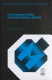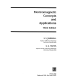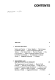### Electromagnetic Fields

#### Course Description

The themes are: Lorentz force, electric field strength, magnetic flux density. Sources: charge and current. Charge at rest: Coulomb s law, Gauss s law, energy and potential in electric field. Dielectrics, conductors, capacitance. Charge in uniform motion: Ohm s law, resistance. Biot-Savart s law, Ampere s circuital law, magnetic materials, energy in magnetic field, inductances, magnetic circuits. Time-dependent fields, Faraday s law, sinusoidal fields. Displacement currents, Maxwell equations, electromagnetic waves.

#### Learning Outcomes

1. Explain the basics laws of electromagnetism (Coulomb, Gauss, Biot-Savart and Faraday)
2. Apply the basic laws of electromagnetism to solve electromagnetic fields problems
3. Classify problems in electromagnetics to static electric, static magnetic, static current and time-varying problems
4. Recognize advantages of application of numerical methods to problems in electromagnetics
5. Apply computations of electromagnetic fields, inductances and capacitances to real-world problems
6. Describe the basic principles of electromechanical energy converison
7. Explain relation between electromagnetic fields and elements of electric circuits
8. Analyze energy transfer and storage in electromagnetic fields

#### Forms of Teaching

Lectures

Involvement in lectures

Independent assignments

preparing for lab classes, homework

Laboratory

Laboratory work

Work with mentor

Lecturers consultations

Continuous Assessment Exam
Laboratory Exercises 0 % 10 % 0 % 10 %
Quizzes 0 % 6 % 0 % 6 %
Mid Term Exam: Written 0 % 30 % 0 %
Final Exam: Written 0 % 30 %
Final Exam: Oral 24 %
Exam: Written 24 % 60 %
Exam: Oral 24 %

#### Week by Week Schedule

1. Lorentz force, electric field strength and magnetic induction, charged particles in electric and magnetic field, macroscopic approach, sources of electromagnetic field, continuity equation.
2. Coulomb's law, electric field strength of point charge, electric dipole, electric field of continuous charge distributions, Gauss's law for electric field.
3. Biot-Savart law, magnetic flux density of current element, magnetic field density of prescribed currents, magnetic dipole, Gauss's law for magnetic field, Ampère's circuital law
4. Faraday's law and definition of voltage, electromagnetic induction in loops at rest, electromagnetic induction in loops in motion, dependence of voltage on path of integration
5. Materials in electric and magnetic field, conductors, insulators, applications of Gauss's law for electric fields, magnetic materials, applications of Gauss's law for magnetic fields
6. Displacement current and Maxwell's equations, boundary conditions at interface separating two media, energy and power in electromagnetic field, Poynting's theorem, time-harmonic fields
7. Static electric field, field equations and scalar electric potential, scalar electric potential of continuous charge distributions, energy, forces and capacitance, calculation of capacitance of condensers
8. Midterm exam
9. Charges at uniform motion, static current field, static magnetic field, vector magnetic potential, energy, forces and inductance, magnetic materials, magnetic circuits
10. Quasi-static electromagnetic field, conductive plate in time-dependent magnetic field - transients, conductive plate in time harmonic magnetic field, conduction of time harmonic current through a plate, skin effect
11. Equations of plane wave. Fundamental characteristics of wave (wave impedance, wavelength, phase constant, phase velocity). Waves in lossless materials.
12. Electromagnetic waves, travelling waves, wave impedance, plane wave, time harmonic plane waves, wave length, phase velocity, polarization of plane waves
13. Time harmonic plane waves in lossy materials, phase and attenuation constant, good insulators, complex permittivity, good conductors, power transmission by plane wave
14. Reflection and refraction of plane wave at plane boundary, laws of reflection and refraction, oblique incidence - perpendicular polarization, oblique incidence - parallel polarization, normal incidence
15. Radiation of electromagnetic field, Hertzian dipole, antenna characteristics, radiation field of thin wire, reciprocity theorem and receiving antennas

#### Study Programmes

Electrical Engineering and Information Technology (study)
(5. semester)

#### Literature

Z. Haznadar, Ž. Štih (1997.), Elektromagnetizam I, Školska knjiga
Z. Haznadar, Ž. Štih (1997.), Elektromagnetizam II, Školska knjiga
Bojan Trkulja (.), Elektromagnetska polja - zadaci za vježbu,
S. Berberović (1998.), Teorijska elektrotehnika - odabrani primjeri, Graphis
Željko Štih, Bojan Trkulja (2010.), Elektromagnetska polja – Magnetostatika,Z. Haznadar, Ž. Štih (2000.), Electromagnetic Fields, Waves and Numerical Methods, IOS PressS.V. Marshall, G.G. Skitek (1990.), Electromagnetic Concepts and Applications, Prentice-HallW.H. Hayt (1988.), Engineering Electromagnetics, McGraw Hill
Dadić, Martin (2013.), Elektromagnetska polja - laboratorijske vježbe, Merkur A.B.D
S. Berberović, Ž. Štih, B. Trkulja (2010.), Elektromagnetska polja - Vremenski promjenjiva EM polja,

#### General

ID 183421
Winter semester
5 ECTS
L3 English Level
L1 e-Learning
60 Lectures
15 Laboratory exercises